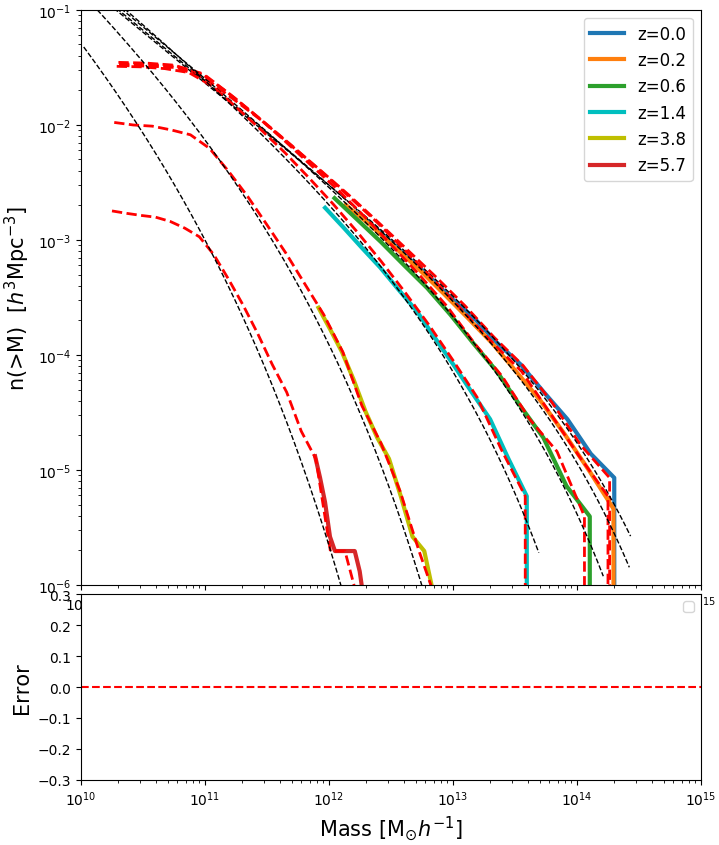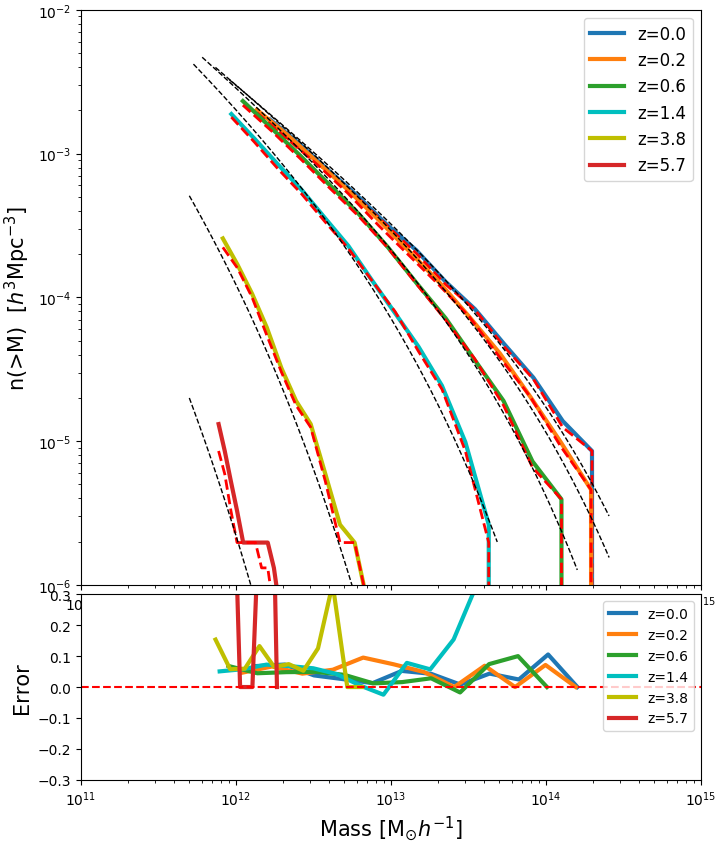# Cosmological Simulations $256^3$ particles, 115 Mpc/h box.

## Cumulative Halo Mass Function

Solid lines correspond to the CHOLLA_PM simulation, red dashed lines correspond to the Gadget simulation and black dashed lines correspond to the model by Warren.

## Case 1:

Gadget: $\epsilon \, = \,9.7 \,kpc/h$

Cholla: $cellSize \, = \, 224.6 \,kpc/h$## Case 2:

For this one the force resolution of Gadget was decreased to match the cell_size used for Cholla

Gadget: $\epsilon \, = \,224.6 \,kpc/h$

Cholla: $cellSize \, = \, 224.6 \,kpc/h$